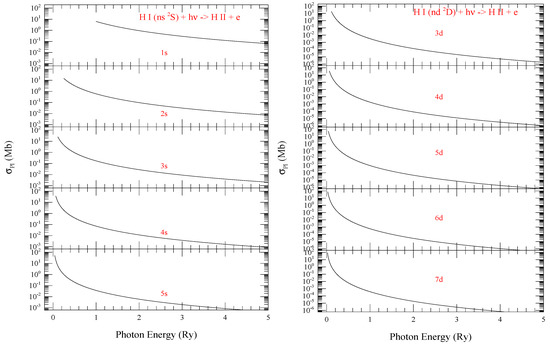Next Article in Journal
Electron Ionization of Size-Selected Positively and Negatively Charged Helium Droplets
Previous Article in Journal
Calculation of the Lowest Resonant States of H and Li by the Complex Absorbing Potential Method
Article

# Photoionization and Electron-Ion Recombination of n = 1 to Very High n-Values of Hydrogenic Ions

Department of Astronomy, The Ohio State University, Columbus, OH 43210, USA
Atoms 2021, 9(4), 73; https://doi.org/10.3390/atoms9040073
Received: 20 August 2021 / Revised: 24 September 2021 / Accepted: 24 September 2021 / Published: 3 October 2021
Single electron hydrogen or hydrogenic ions have analytical forms to evaluate the atomic parameters for the inverse processes of photoionization and electron-ion recombination (H I + h$\nu ↔$ H II + e) where H is hydrogen. Studies of these processes have continued until the present day (i) as the computations are restricted to lower principle quantum number n and (ii) to improve the accuracy. The analytical expressions have many terms and there are numerical instabilities arising from cancellations of terms. Strategies for fast convergence of contributions were developed but precise computations are still limited to lower n. This report gives a brief review of the earlier precise methodologies for hydrogen, and presents numerical tables of photoionization cross sections (${\sigma }_{PI}$), and electron-ion recombination rate coefficients (${\alpha }_{RC}$) obtained from recombination cross sections (${\sigma }_{RC}$) for all n values going to a very high value of 800. ${\sigma }_{PI}$ was obtained using the precise formalism of Burgess and Seaton, and Burgess. ${\alpha }_{RC}$ was obtained through a finite integration that converge recombination exactly as implemented in the unified method of recombination of Nahar and Pradhan. Since the total electron-ion recombination includes all levels for n = 1–∞, the total asymptotic contribution of n = 801–∞, called the top-up, is obtained through a ${n}^{-3}$ formula. A FORTRAN program “hpxrrc.f” is provided to compute photoionization cross sections, recombination cross sections and rate coefficients for any $nl$. The results on hydrogen atom can be used to obtain those for any hydrogenic ion of charge z through z-scaling relations provided in the theory section. The present results are of high precision and complete for astrophysical modelings. View Full-Text
Show FiguresFigure 1

MDPI and ACS Style

Nahar, S.N. Photoionization and Electron-Ion Recombination of n = 1 to Very High n-Values of Hydrogenic Ions. Atoms 2021, 9, 73. https://doi.org/10.3390/atoms9040073

AMA Style

Nahar SN. Photoionization and Electron-Ion Recombination of n = 1 to Very High n-Values of Hydrogenic Ions. Atoms. 2021; 9(4):73. https://doi.org/10.3390/atoms9040073

Chicago/Turabian Style

Nahar, Sultana N. 2021. "Photoionization and Electron-Ion Recombination of n = 1 to Very High n-Values of Hydrogenic Ions" Atoms 9, no. 4: 73. https://doi.org/10.3390/atoms9040073

Find Other Styles
Note that from the first issue of 2016, MDPI journals use article numbers instead of page numbers. See further details here.

1